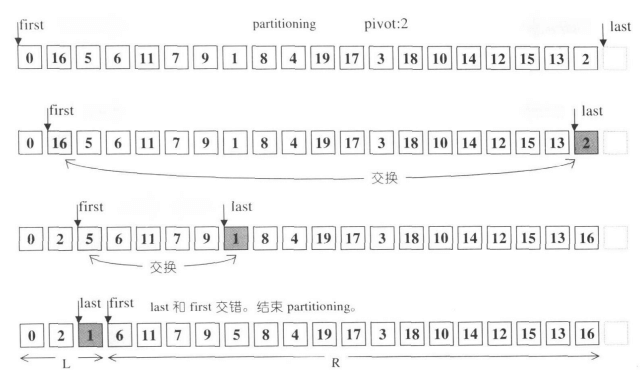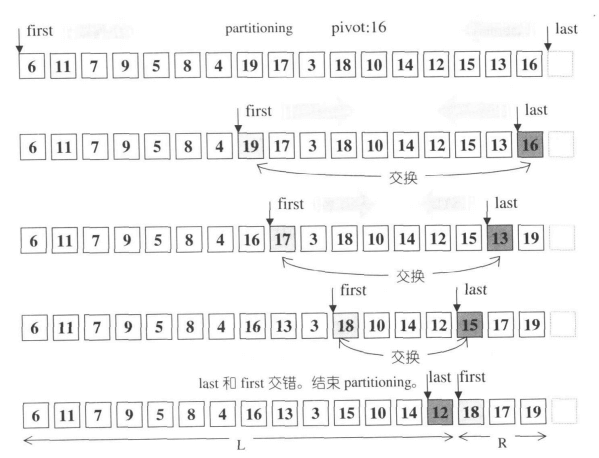β

# 谈谈 STL 中的 std::sort

C++ 的标准模板库有一个很霸气的解读：「标准模板库里的任意算法、数据结构，你找不到一个实现，在所有的情况下都优于标准模板库的实现；否则，它就应该进入标准模板库」。因此，对于排序问题来说，C++ 里的标准模板库中的 ``` std::sort ``` 可想而知是一个在绝大多数情况下都能达到极限性能的排序算法。

## ``` std::sort ```

``template <class RandomAccessIterator>inline void sort(RandomAccessIterator first, RandomAccessIterator last) {    if (first != last) {        __introsort_loop(first, last, value_type(first), __lg(last - first) * 2);        __final_insertion_sort(first, last);    }}``

## ``` __introsort_loop ```

``template <class RandomAccessIterator, class T, class Size>void __introsort_loop(RandomAccessIterator first,                      RandomAccessIterator last, T*,                      Size depth_limit) {    while (last - first > __stl_threshold) {        if (depth_limit == 0) {            partial_sort(first, last, last);            return;        }        --depth_limit;        RandomAccessIterator cut = __unguarded_partition          (first, last, T(__median(*first, *(first + (last - first) / 2),                                   *(last - 1))));        __introsort_loop(cut, last, value_type(first), depth_limit);        last = cut;    }}``

### ``` __stl_threshold ```

``` __stl_threshold ``` 是一个预定义的宏，它对应 前文 提到的超参数。当左闭右开区间的长度不大于该超参数时，可以认为序列基本有序，于是退出内省式排序，转向插入排序。

### ``` if (depth_limit == 0) ``` 与 ``` --depth_limit ```

``template <class RandomAccessIterator, class T, class Compare>void __partial_sort(RandomAccessIterator first, RandomAccessIterator middle,                    RandomAccessIterator last, T*, Compare comp) {    make_heap(first, middle, comp);    for (RandomAccessIterator i = middle; i < last; ++i)        if (comp(*i, *first))            __pop_heap(first, middle, i, T(*i), comp, distance_type(first));    sort_heap(first, middle, comp);}template <class RandomAccessIterator, class Compare>inline void partial_sort(RandomAccessIterator first,                         RandomAccessIterator middle,                         RandomAccessIterator last, Compare comp) {    __partial_sort(first, middle, last, value_type(first), comp);}``

### 递归结构

``qsort(first, last):    cut = partition(first, last, pivot)  // pivot is picked in [first, last)    qsort(cut + 1, last)    qsort(first, cut)``

``qsort(first, last):    while (valid) {        cut = partition(first, last, pivot)        qsort(cut + 1, last)        last = cut    }``

### pivot 的选择

``__median(*first, *(first + (last - first) / 2), *(last - 1))``

### ``` __unguarded_partition ```

``template <class RandomAccessIterator, class T>RandomAccessIterator __unguarded_partition(RandomAccessIterator first,                                           RandomAccessIterator last,                                           T pivot) {    while (true) {        while (*first < pivot) ++first;      // 1.2        --last;                              // 2.1        while (pivot < *last) --last;        // 2.2        if (!(first < last)) return first;   // 3.        iter_swap(first, last);              // 4.        ++first;                             // 1.1    }}``

(1.1) 和 (1.2) 配合，将 ``` first ``` 迭代器后移，指向第一个不小于主元的元素。(2.1) 和 (2.2) 配合，将 ``` last ``` 迭代器前移，指向第一个不大于主元的元素。在 (3) 处的判断，若第一个不小于主元的元素不先序于第一个不大于主元的元素，则说明分割已经完毕，返回第一个不小于主元的元素的位置，即 ``` first ``` 的当前值；否则，在 (4) 处交换 ``` first `````` last ``` 指向元素的值。参考《STL 源码剖析》的图示：• 函数没有进行任何边界判断而不会陷入死循环的原因是 ``` pivot `````` [first, last) ``` 内的元素，因此在循环中 ``` first `````` last ``` 必然会破坏先序关系（不一定是交错）。避免边界判断，又节省了不少比较开销。
• 不同于标准的 partition 返回主元的分割位置， ``` __unguarded_partition ``` 返回的是第一个不小于主元的元素位置。由于在 ``` [first, last) ``` 长度足够小时，函数会退出并交由插入排序实现，这种设计并不会影响算法的正确性。

## ``` __final_insertion_sort ```

``` __introsort_loop `````` [first, last) ``` 调整为基本有序后，就会转而进入插入排序。

``template <class RandomAccessIterator>void __final_insertion_sort(RandomAccessIterator first,                            RandomAccessIterator last) {    if (last - first > __stl_threshold) {        __insertion_sort(first, first + __stl_threshold);        __unguarded_insertion_sort(first + __stl_threshold, last);    } else {        __insertion_sort(first, last);    }}``

1. ``` __insertion_sort `````` __unguarded_insertion_sort ``` 分别是什么？它们与标准的插入排序有什么区别？各自的适用条件又是什么？
2. 在区间长度较长时，为什么要将前 ``` __stl_threshold ``` 个元素单独处理？
3. 为什么要对区间长度的大小进行划分？这与 ``` __introsort_loop `````` while ``` 循环的区间长度限制有什么联系？

### 插入排序的实现

``template <class RandomAccessIterator>void insertion_sort(RandomAccessIterator first, RandomAccessIterator last) {    if (not(first < last)) return;    for (RandomAccessIterator i = first + 1; i != last; ++i) {  // 1.        auto key = *i;        RandomAccessIterator j;        for (j = i - 1; first <= j and key < *j; --j) {         // 2.            *(j + 1) = *j;                                      // 3.        }        *(j + 1) = key;                                         // 4.    }}``

``template <class RandomAccessIterator, class T>void __unguarded_linear_insert(RandomAccessIterator last, T value) {    RandomAccessIterator next = last;    --next;    while (value < *next) {        *last = *next;        last = next;        --next;    }    *last = value;}``

``` __unguarded_linear_insert ``` 函数模板的实现如其名称所展现的那样，它在对 ``` next ``` 迭代器的自减中，没有检查 ``` next ``` 迭代器是否向左超越边界。更有甚者，它根本无需输入左边界，而只需输入右边界迭代器和带插入元素的值即可；这也意味着， ``` __unguarded_linear_insert ``` 不是完整的插入排序的实现。事实上，它只完成了原版插入排序中 (2)(3)(4) 的部分功能。

``template <class RandomAccessIterator, class T>inline void __linear_insert(RandomAccessIterator first,                            RandomAccessIterator last, T*) {    T value = *last;    if (value < *first) {        copy_backward(first, last, last + 1);        *first = value;    } else {        __unguarded_linear_insert(last, value);    }}``

``` __linear_insert ``` 函数模板的意图是将 ``` last ``` 所指向的元素插入到正确位置，这里蕴含的前提假设是 ``` [first, last) ``` 区间的元素是已经排好序的 。在这一假设下，若 ``` *last < *first ``` ，则毫无疑问， ``` last ``` 指向的元素应当插入在上述区间的最前面，因此有 ``` std::copy_backward ``` ；若不满足条件判断，则在 ``` [first, last) ``` 之间必然存在不大于 ``` value ``` 的元素（比如至少 ``` *first ``` 是这样），因此可以调用 ``` __unguarded_linear_insert ``` 来解决问题，而不必担心在 ``` __unguarded_linear_insert `````` next ``` 迭代器向左越界。对于 ``` *last < *first ``` 的情况， ``` __linear_insert `````` last - first - 1 ``` 次比较和交换操作变成了一次 ``` std::copy_backward ``` 操作，相当于节省了 ``` last - first - 1 ``` 次比较操作。

``template <class RandomAccessIterator>void __insertion_sort(RandomAccessIterator first, RandomAccessIterator last) {    if (first == last) return;    for (RandomAccessIterator i = first + 1; i != last; ++i)        __linear_insert(first, i, value_type(first));}``

``template <class RandomAccessIterator, class T>void __unguarded_insertion_sort_aux(RandomAccessIterator first,                                    RandomAccessIterator last, T*) {    for (RandomAccessIterator i = first; i != last; ++i)        __unguarded_linear_insert(i, T(*i));}template <class RandomAccessIterator>inline void __unguarded_insertion_sort(RandomAccessIterator first,                                RandomAccessIterator last) {    __unguarded_insertion_sort_aux(first, last, value_type(first));}``

``` __unguarded_insertion_sort_aux ``` 是一个简单的辅助函数，其目的只是获取模板参数 ``` T ``` ，以便正确调用 ``` __unguarded_linear_insert `````` __unguarded_insertion_sort ``` 没有边界检查，因此它一定比 ``` __insertion_sort ``` 要快。但由于 ``` __unguarded_insertion_sort_aux ``` 会从 ``` first ``` 开始调用 ``` __unguarded_linear_insert ``` ；因此使用 ``` __unguarded_insertion_sort ``` 的条件比 ``` __unguarded_linear_insert ``` 更加严格。它必须保证以下假设成立： ``` first ``` 左边的有效位置上，存在不大于 ``` [first, last) ``` 中所有元素的元素

### 回答三个问题

• ``` __insertion_sort ``` 实现了标准的插入排序的功能。但由于内部使用了 ``` __linear_insert `````` __unguarded_linear_insert ``` 内部函数，其效率比标准的插入排序效率要高。
• ``` __unguarded_insertion_sort ``` 基本上也实现了插入排序的思想，但它实现的功能比标准的插入排序稍弱。它要求在 ``` first ``` 左边的有效位置上，存在不大于 ``` [first, last) ``` 中所有元素的元素。

• ``` __unguarded_insertion_sort ``` 的效率比 ``` __insertion_sort ``` 高，因此我们应该尽可能对更多的元素使用 ``` __unguarded_insertion_sort ``` 。但使用它是有前提的，因此至少对最头部的一些元素，我们不能使用 ``` __unguarded_insertion_sort ```
• 但这只是部分回答了第二个问题；因为我们仍然没有说明为什么对头部 ``` __stl_threshold ``` 个元素之外的元素使用 ``` __unguarded_insertion_sort ``` 是安全的。也即我们需要证明：头部 ``` __stl_threshold ``` 个元素中一定存在不小于区间 ``` [first + __stl_threshold, last) ``` 中所有元素的元素。

• ``` last - first > __stl_threshold ``` 不成立，则事实上 ``` __introsort_loop ``` 不会对序列做任何实际操作——这是由内省式排序 ``` while ``` 循环的条件确保的。这种情况下，不能使用 ``` __unguarded_insertion_sort ``` ，而是要使用具有完整排序能力的 ``` __insertion_sort ``` 来执行最终的插入排序操作。

### 完整回答第二个问题

1. 因递归切分，导致 ``` last - first > __stl_threshold ``` 不成立而退出。
2. 因递归过深，调用堆排序而退出。

## 结语

C++ 的标准模板库有一个很霸气的解读：「标准模板库里的任意算法、数据结构，你找不到一个实现，在所有的情况下都优于标准模板库的实现；否则，它就应该进入标准模板库」。因此，对于排序问题来说，C++ 里的标准模板库中的 ``` std::sort ``` 可想而知是一个在绝大多数情况下都能达到极限性能的排序算法。